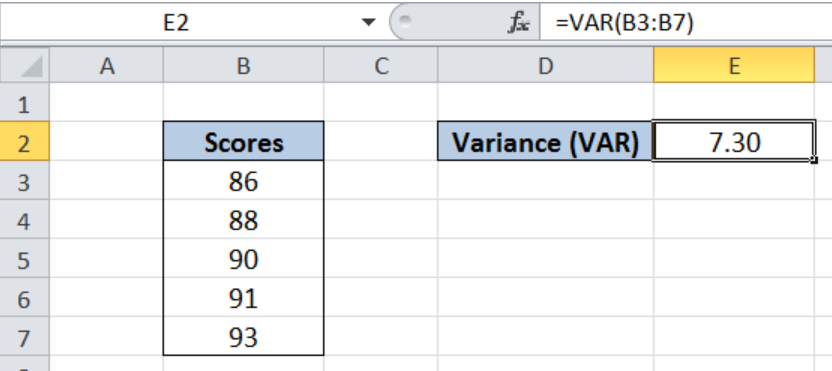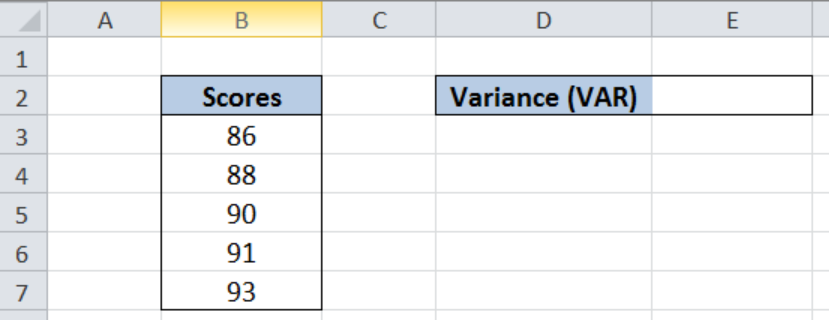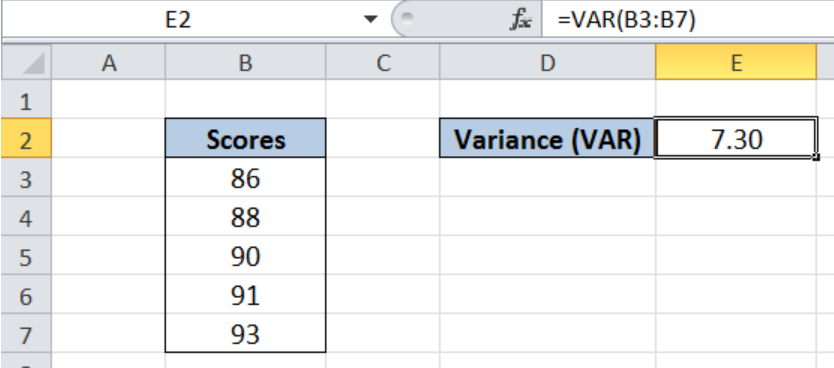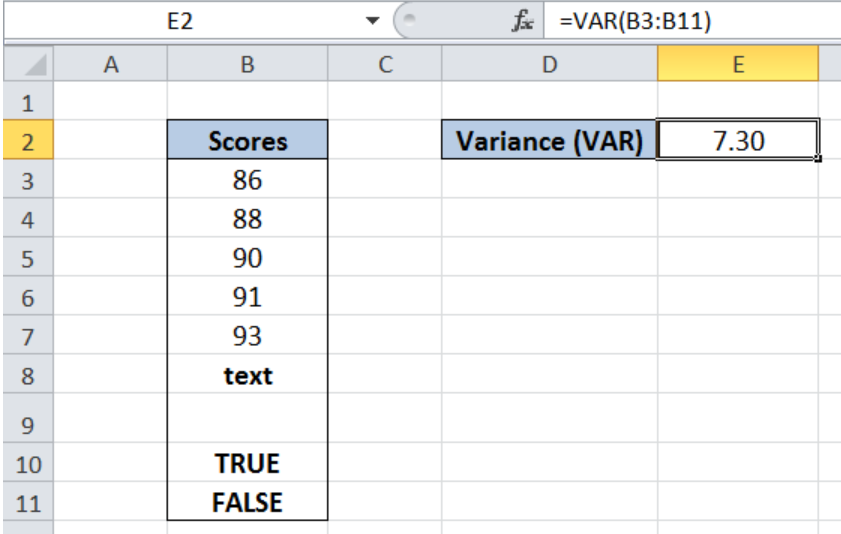Get instant live expert help with Excel or Google Sheets“My Excelchat expert helped me in less than 20 minutes, saving me what would have been 5 hours of work!”

#### Post your problem and you’ll get Expert help in seconds.

Your message must be at least 40 characters
Our professional Expert are available now. Your privacy is guaranteed.

# Excel VAR Function

The VAR function in Excel estimates the variance of a sample of data, while ignoring empty cells, text and logical values.  This step by step tutorial will assist all levels of Excel users in the usage and syntax of the VAR function.Figure 1. Final result: Excel VAR function

Final formula: `=VAR(B3:B7)`

## Syntax of the VAR Function

`=VAR(number1,[number2],...])`

• number1 – any number, array or cell reference representing a sample of a population whose variance we want to calculate
• Only number1 is required; succeeding numbers are optional

## Setting up the Data

We have here a list of Scores in column B.  We want to calculate the variance of our data in cell E2.Figure 2. Sample data to calculate variance using VAR function

## Calculate variance using VAR function

In order to calculate the variance of the sample of data, we follow these steps:

Step 1. Select cell E2

Step 2. Enter the formula: `=VAR(B3:B7)`

Step 3. Press Enter

The range B3:B7 contains our data.  The VAR formula calculates the variance and returns 7.30 in cell E2.Figure 3. Output: Calculate variance using VAR function

## Notes:

• VAR function ignores the following: empty cells, logical values {TRUE or FALSE}, text values
• In order to evaluate logical values or text, we use the VARA function
• We use VAR when we have a sample of the data; if our data represents the entire population, we use VARP

Below example shows four more cells are added into our list of scores: a text value, an empty cell, and logical values TRUE and FALSE.  Note that the resulting variance calculated by the VAR function is still 7.30, which is the same as in our previous example.Figure 4. Output: VAR function ignores blank cells, text and logical values

Most of the time, the problem you will need to solve will be more complex than a simple application of a formula or function. If you want to save hours of research and frustration, try our live Excelchat service! Our Excel Experts are available 24/7 to answer any Excel question you may have. We guarantee a connection within 30 seconds and a customized solution within 20 minutes.

### Did this post not answer your question? Get a solution from connecting with the expert.Another blog reader asked this question today on Excelchat:
Solution examplesUse the Vlookup Function to complete the "employee" column of table 2. Use "job Id" from table 2 as your lookup_value(s) and table 1 as your reference.
Solved by C. H. in 16 minsIf a cell in another sheet is populated I need a vlookup done. If the cell is not populated I need the cell to return blank.
Solved by T. D. in 60 minsI am trying to make a chart that turns a week range red if nothing is entered in the range. If something is entered then I would like it to turn green. Please Help
Solved by E. U. in 43 minsI need a check box to show/hide an answer of an if function
Solved by Z. U. in 23 minsI need a formula to compare the data in two columns and then export the mismatched data in the 3rd column
Solved by S. Q. in 20 mins## Subscribe to Excelchat.coAnother blog reader asked this question today on Excelchat: